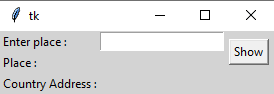# GUI application to search a country name from a given state or city name using Python

• Last Updated : 04 Dec, 2021

In these articles, we are going to write python scripts to search a country from a given state or city name and bind it with the GUI application. We will be using the GeoPy module. GeoPy modules make it easier to locate the coordinates of addresses, cities, countries, landmarks, and Zipcode.

Before starting we need to install the GeoPy module, so let’s run to this command on your terminal.

`pip install geopy`

Approach:

• Import module
• Use Nominatim API to access the corresponding to set of coordinates
• geocode() to get the location of a given place

The GUI would look like below:Note: Nominatim uses OpenStreetMap data to find locations on Earth by name and address (geocoding).

Below is the Implementation:

## Python3

 `from` `geopy.geocoders ``import` `Nominatim`  `geolocator ``=` `Nominatim(user_agent ``=` `"geoapiExercises"``)``location ``=` `geolocator.geocode(``"Delhi"``)``print``(``"Country Name: "``, location)`

Output:

Country Name:  Delhi, Kotwali Tehsil, Central Delhi, Delhi, 110006, India

Application for Searching Country from given city/state with Tkinter: This Script implements the above Implementation into a GUI.

## Python3

 `# importing the modules``from` `geopy.geocoders ``import` `Nominatim``from` `tkinter ``import` `*``from` `tkinter ``import` `messagebox` `def` `getinfo():``    ``geolocator ``=` `Nominatim(user_agent ``=` `"geoapiExercises"``)``    ``place ``=` `e.get()``    ``place_res.``set``(place)``    ``location ``=` `geolocator.geocode(place)``    ``res.``set``(location)` `# object of tkinter``# and background set for light grey``master ``=` `Tk()``master.configure(bg ``=` `'light grey'``)` `# variable Classes in tkinter``place_res ``=` `StringVar();``res ``=` `StringVar();` `# creating label for each information``# name using widget Label``Label(master, text ``=` `"Enter place :"` `,``      ``bg ``=` `"light grey"``).grid(row ``=` `0``, sticky ``=` `W)``Label(master, text ``=` `"Place :"` `,``      ``bg ``=` `"light grey"``).grid(row ``=` `1``, sticky ``=` `W)``Label(master, text ``=` `"Country Address :"` `,``      ``bg ``=` `"light grey"``).grid(row ``=` `2``, sticky ``=` `W)` `# creating label for class variable``# name using widget Entry``Label(master, text ``=` `"", textvariable ``=` `place_res,``      ``bg ``=` `"light grey"``).grid(row ``=` `1``, column ``=` `1``, sticky ``=` `W)``Label(master, text ``=` `"", textvariable ``=` `res,``      ``bg ``=` `"light grey"``).grid(row ``=` `2``, column ``=` `1``, sticky ``=` `W)` `e ``=` `Entry(master)``e.grid(row ``=` `0``, column ``=` `1``)` `# creating a button using the widget ``# Button that will call the submit function``b ``=` `Button(master, text ``=` `"Show"``, command ``=` `getinfo )``b.grid(row ``=` `0``, column ``=` `2``, columnspan ``=` `2``,``       ``rowspan ``=` `2``, padx ``=` `5``, pady ``=` `5``)` `mainloop()`

Output:

My Personal Notes arrow_drop_up